### IMO Shortlist 1974 problem 3

Kvaliteta:
Avg: 0,0
Težina:
Avg: 0,0
Let$P(x)$ be a polynomial with integer coefficients. We denote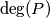$\deg(P)$ its degree which is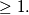$\geq 1.$ Let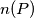$n(P)$ be the number of all the integers$k$ for which we have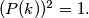$(P(k))^{2}=1.$ Prove that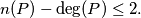$n(P)- \deg(P) \leq 2.$
Izvor: Međunarodna matematička olimpijada, shortlist 1974